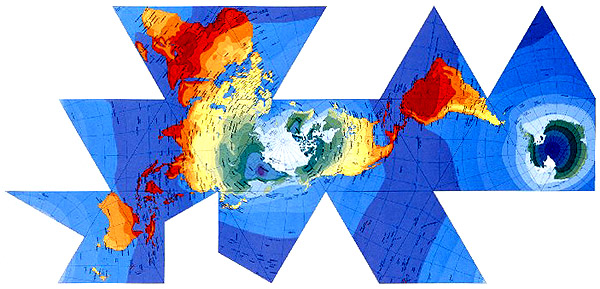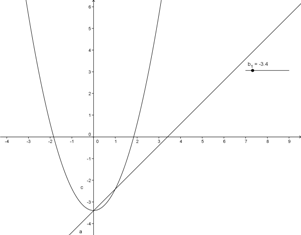# Sand BoxAllPages RecentChanges Links to this page Edit this page Search Entry portal Advice For New Users

$\frac{\pi}{\sum_{n=0}^\infty{(-1)^n\over{(2n+1)}}}$

$-1=e^{i\pi}=(e^\pi)^i$ would be transcendental

...

This is a place you can play. Try anything you like here, just remember, things built on sand don't last !!

 Here is a table of words to see how it works

Bold italic

Make the rest of the line red.

# Section

• Bullet list
• Second item
• Third (red) item

## Equations:

using wiki markup:

$\int_0^\infty\frac{a+b}{c}dx$
$|\b{x}|$ $|x|$

or as anti-aliased gif-file from TechWriter:DOC:quaternions.html

DOC:test.pdf

DOC:Circles.ggb